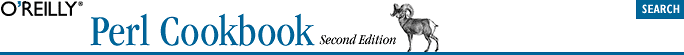home | O'Reilly's CD bookshelfs | FreeBSD | Linux | Cisco | Cisco Exam## 11.15. Coping with Circular Data Structures Using Weak References

### 11.15.2. Solution

Convert all internal references within the data structure into weak references so they don't increment the reference count.

### 11.15.3. Description

Perl's memory management system relies on an underlying reference count to know when to reclaim memory. In practice, this works fairly well except for one particular situation: when a variable directly or indirectly points at itself. Consider:

```{
my (\$a, \$b);
(\$a, \$b) = \(\$b, \$a);   # same as (\\$b, \\$a);
}```

The two underlying scalars that \$a and \$b represent each start out with a reference count of one apiece in the first line of the block. In the second line, those scalars are each initialized to contain a reference to the other variable; \$a points to \$b and vice versa. Saving a reference increments the underlying reference count on the scalars, so now both refcounts are set to two. As the block exits and those lexical variables become unreachable (by name), both refcounts are decremented by one, leaving one in each—forever. Since the refcounts can never reach zero, memory used by those two underlying scalars will never be reclaimed. You'll leak two scalars every time that block executes; if it's a loop or a subroutine, you could eventually run out of memory.

```use Devel::Peek;
\$a = 42;
\$b = \\$a;
Dump \$a;```

produces this output:

```SV = IV(0xd7cc4) at 0xd72b8
REFCNT = 2
FLAGS = (IOK,pIOK)
IV = 42```

The important thing to notice there is that the refcount is two. That's because the scalar can be reached two ways: once via the variable named \$a, and then again through dereferencing \$b using \$\$b.

You can produce the same condition, even without using another variable:

`{ my \$a; \$a = \\$a; }`

This most often occurs when creating a data structure whose elements contain references that directly or indirectly point back to the initial element. Imagine a circular linked list—a ring data structure.

```\$ring = {
VALUE => undef,
NEXT  => undef,
PREV  => undef,
};
\$ring->{NEXT} = \$ring;
\$ring->{PREV} = \$ring;```

The underlying hash has an underlying refcount of three, and undef fing \$ring or letting it go out of scope will decrement that count only by one, resulting in a whole hash full of memory irrecoverable by Perl.

To address this situation, Perl introduced in its v5.6 release the concept of weak references. A weak reference is just like any other regular reference (meaning a "hard" reference, not a "symbolic" one) except for two critical properties: it no longer contributes to the reference count on its referent, and when its referent is garbage collected, the weak reference itself becomes undefined. These properties make weak references perfect for data structures that hold internal references to themselves. That way those internal references do not count toward the structure's reference count, but external ones still do.

Here's how you would apply weak references to the ring example just given:

```use Scalar::Util qw(weaken);

\$ring = {
VALUE => undef,
NEXT  => undef,
PREV  => undef,
};
\$ring->{NEXT} = \$ring;
\$ring->{PREV} = \$ring;
weaken(\$ring->{NEXT});
weaken(\$ring->{PREV});```

In Recipe 13.13, we show how to create a circular-linked list data structure that won't leak memory by employing an elaborate trick using a dummy head node and an object-oriented device called a destructor. With weak references, the code becomes much simpler. Here's the same algorithm as that recipe uses, but here using weak references to address the memory-leak issue.

```use Scalar::Util qw(weaken);

my \$COUNT = 1000;
for (1..20) {
my \$ring = node(100_000 + \$_);
for my \$value (1 .. \$COUNT) {
insert_value(\$ring, \$value);
}
}

# return a node
sub node(\$) {
my (\$init_value) = @_;
my \$node  = { VALUE => \$init_value };
\$node->{NEXT} = \$node->{PREV} = \$node;
weaken(\$node->{NEXT});
weaken(\$node->{PREV});
return \$node;
}

# \$node = search_ring(\$ring, \$value) : find \$value in the ring
# structure in \$node
sub search_ring {
my (\$ring, \$value) = @_;
my \$node = \$ring->{NEXT};
while (\$node != \$ring && \$node->{VALUE} != \$value) {
\$node = \$node->{NEXT};
}
return \$node;
}

# insert_value( \$ring, \$value ) : insert \$value into the ring structure
sub insert_value {
my (\$ring, \$value) = @_;
my \$node = { VALUE => \$value };
weaken(\$node->{NEXT} = \$ring->{NEXT});
weaken(\$ring->{NEXT}->{PREV} = \$node);
weaken(\$ring->{NEXT} = \$node);
weaken(\$node->{PREV} = \$ring);
++\$ring->{COUNT};
}

# delete_value( \$ring, \$value ) : delete a node from the ring
# structure by value
sub delete_value {
my (\$ring, \$value) = @_;
my \$node = search_ring(\$ring, \$value);
return if \$node =  = \$ring;
\$ring->delete_node(\$node);
}

# delete a node from the ring structure
sub delete_node {
my (\$ring, \$node) = @_;
weaken(\$node->{PREV}->{NEXT} = \$node->{NEXT});
weaken(\$node->{NEXT}->{PREV} = \$node->{PREV});
--\$ring->{COUNT};
}```

Every time we store a reference to part of the data structure within that same structure, we weaken the reference so it doesn't count toward the reference count. Otherwise our program's in-core memory footprint would have grown terrifically. You can watch that happen by adding:

`system("ps v\$\$");`

within the loop on systems that support the ps(1) program. All it takes to trigger the leak is not weakening any of the four assignments in the insert_value function just shown.